Mathematical Model of Compost Pile Temperature Prediction | OMICS International
Journal of Environmental & Analytical Toxicology

Like us on:

# Mathematical Model of Compost Pile Temperature Prediction

 El-Sayed G Khater*, Adel H Bahnasawy and Samir A Ali Agricultural Engineering Department, Faculty of Agriculture, Benha University, 13736, Egypt Corresponding Author : El-Sayed G. Khater Agricultural Engineering Department Faculty of Agriculture, Benha University 13736, Egypt Tel: +20132467034 E-mail: [email protected] Received September 02, 2014; Accepted Septemebr 19, 2014; Published September 22, 2014 Citation: Khater EG, Bahnasawy AH, Ali SA (2014) Mathematical Model of Compost Pile Temperature Prediction. J Environ Anal Toxicol 4:242. doi: 10.4172/2161-0525.1000242 Copyright: © 2014 Khater EG, et al. This is an open-access article distributed under the terms of the Creative Commons Attribution License, which permits unrestricted use, distribution, and reproduction in any medium, provided the original author and source are credited.

Visit for more related articles at Journal of Environmental & Analytical Toxicology

#### Abstract

A mathematical model of heat balance was developed to predict the compost temperature during the maturation stage. The components of the energy balance of the compost pile which include: heat gained (heat generation and solar radiation) and heat lost (radiation, evaporation, convection, and conduction) at different ambient temperatures. The model was able to predict the pile temperature at different ambient temperatures (15, 20, 25, 30 and 35°C) and different airflow rates (0.7, 1.1 and 1.5 mg air s-1 kg-1 dry matter). The results showed that the pile temperature increases with increasing ambient temperature and it decreases with increasing airflow rates, where, as the ambient temperature increased from 15 to 35°C, the pile temperature increased from 33.40 to 37.41°C, and when the airflow rates increased from 0.7 to 1.5 mg air s-1 kg-1 dry matter, the pile temperature decreased from 34.40 to 32.39°C. The pile temperature increased slightly and reached a maximum value at day 14. It indicates that the net energy gained to the pile increases with increasing ambient temperature, meanwhile, the heat lost decreases with increasing ambient temperature. The model results indicated that the predicted daily temperature was in a reasonable agreement with those measured ones and other data in literature (Barrena et al. and Ahn et al.) at different ambient temperatures and airflow rates, where, it ranged from 30.30 to 73.40°C, while it was from 18.0 to 71.0°C experimentally during the whole period of compost maturation.

 Keywords Heat balance; Mathematical model; Pile temperature; Compost Introduction Composting is becoming an attractive and environmentally friendly technology to treat and recycle organic solid wastes, and its use is increasing in developed countries. From the technical and scientific point of view, composting is an extraordinarily complex process in which mass and energy transfer phenomena and microbiological degradation and non-steady state conditions coexist. Composting is an aerobic process, which requires oxygen to stabilize the organic wastes, optimal moisture and porosity. Temperature, oxygen and moisture are often selected as the control variables in the composting process, because they can be simply determined and they are the most significant parameters in controlling the process . Temperature is one of the most important factors in maintaining a proper composting system. The estimation of the thermal balance in the composting process is important in understanding bioreactor dynamics and providing proper temperature control. Investigations of optimal operational factors and proper control of the composting process have been reported by several researchers [1-3]. In a few previous works, other relevant studies for the prediction of temperature in a composting process have been carried out. Some of studies have focused on the study of surface compost temperatures of composting heaps and their relation to core temperatures  in an aerated active composting pile, with the aim of inferring pathogen deactivation, since important temperature gradients have been observed in large composting masses . Other related works have studied the temperature effect on the biodegradation kinetics  or the effect of turning on the temperature and oxygen profiles in composting . Recently, the calculation of the heat generation fluxes was assumed well known and the papers aimed to characterize the heat transfers. However, both the way the heat generation flux was calculated, and the values used, or fitted from modelling of heat transfers, for the heat generation coefficient, varied from one author to the others [1,8-16]. Thus,  reported that the heat generation coefficient varied between 17.8 and 24.7 kJ/g DM removed and between 304 and 448 kJ/mole O2 consumed. Such variations can justify since the enthalpy of formation and the stoichiometry of bio-oxidation of every waste may depend on its biochemical composition. However, as mentioned above, the heat release is often calculated on basis of thermal balance assuming heat transfers are valid whereas determine heat transfer coefficients is rather complex and that uncertainties regards heat transfers still remain. In windrow,  estimated losses by evaporation, radiation and convection to respectively 70, 20 and 10%. Thus, natural convection including evaporation and transfers between composting material and gas surrounding material were recognized as the main contributors to heat loss in detriment of radiation [13,19] also studied the influences size and shape of windrow upon both heat losses, by convection and radiation, and material temperature. One of the main problems that hinder production compost is the difficulty to control the compost temperature, therefore, the main aim of this work is to develop a mathematical model of heat balance to predict the compost temperature during the maturation stage to help in a proper compost production. Theoretical Approach The following assumptions were taken into account while developing the model: a. The system maintains a constant pressure. b. Gas flow rates of the air at compost pile inlet and outlet are equal. c. The substrate is a homogeneous mixture of uniform composition. d. Elementary composition of organic matter in the substrate (carbon, hydrogen, oxygen and nitrogen) is known at the beginning of the process. e. The composting rate is expressed as the rate of organic matter degradation. Equation 1 and 2 present the heat balance components of the compost pile as shown in Figure 1: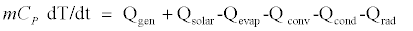(1)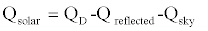(2) Heat generated during the maturation stage can be described by the following equation :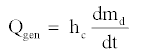(3) The degradation of dry matter was modeled after the equation presented by  which is: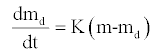(4) Radiation emitted by the Sun travels through the vacuum of space unaltered. The percentage of energy associated with certain bandwidths of solar radiation emitted from a blackbody at 5800K . To determine the amount of incoming extraterrestrial radiation, the following equations can be used: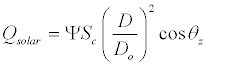(5)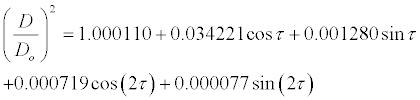(6)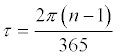(7) The solar zenith (θz) is the angle formed by the pile normal and direct incident beam radiation and this angle varies with the time of day, the time of year and the geographical position of the pile. The solar zenith is given by the following equations [23,24]: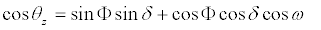(8)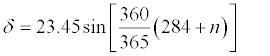(9)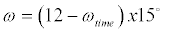(10)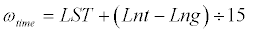(11) The reflectivity of solar radiation varies with the angle of incidence of the incoming radiation will be negligible. Longwave sky radiation can be calculated using the following equation [25,26].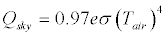(12) The average emittance of the atmosphere terms can be calculated according to the following equations :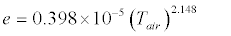(13) Convective heat loss is caused by the enthalpy differences between air entering and exiting the pile. It can be described by the following equation :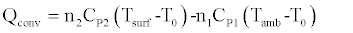(14) Standard molar composition of air entering the pile was used (21% of O2 and 79% of N2), whereas the average molar composition of air exiting the pile was approximately 15% of O2, 79% of N2 and the rest (6%) is assumed to be CO2. Values of CP1 and CP2 can be calculated according to the physical properties of these compounds. Conductive and radiation heat loss terms can be calculated according to the following equations, respectively :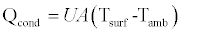(15)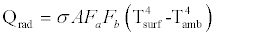(16) Surface temperature (Tsurf) is a crucial parameter for the simulation of temperature profiles since it determines the heat loss by convection, conduction and radiation mechanisms. To estimate Tsurf, a local calorific balance was carried out, taking into account that given a heat exchange area and under pseudo-steady state conditions, the heat internally transferred from the core to the surface of the pile must be equal to the heat loss in the surface (conduction and radiation terms) as shown in the following equation :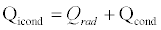(17)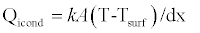(18) Energy loss by water evaporation can be calculated by the equation 19 . This value must be considered as specific to this waste, since water loss by evaporation is mainly dependent on the compost temperature and porosity. Although water evaporation is the main contribution to maintain and control temperature in aerated active thermophilic composting processes .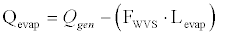(19) The gaseous flow going out from the composting material was assumed saturated in vapour and the mass of water going out from material as vapour was calculated as :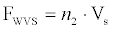(20) The content in vapour in saturated gas (Vs) was calculated to mathematical regression of psychometric charts between 10 and 110°C :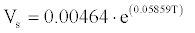(21) The latent heat of evaporation was obtained by applying: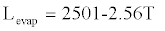(22) All computational procedures of the model were carried out using Excel spreadsheet. The computer program was devoted to heat balance for predicting the temperature of compost pile. Figure 2 shows the flowchart of the model. Table 1 shows the initial (before maturation) and final (after maturation) characteristics of the maturation pile. Table 2 shows the parameters used in the model. The parameters used in the model that were obtained from the literature are listed in Table 2. Experimental Procedures The experiment was carried out at the Compost Unit at Experimental Research Station at the Faculty of agriculture, Moshtohor, Benha University. Some of agricultural wastes are used for compost production, these wastes are cattle manure, herbal plants residues and sugar cane plants residues. The raw materials properties that used in the manufacturing the compost are listed in Table 3. The mixture of raw materials was placed in three piles of 2.20 m wide, 1.70 m height and 12.00 m long. Two sets of PVC perforated pipes φ 5 cm 2.00 m long were placed horizontally parallel two the pile. Six pipes with same diameter were fixed vertically and distribution at 2.00 m distance (Figure 3). Temperature was recorded at different depths (40, 80 and 120 cm horizontally and 40 cm distance vertically) using a thermo-couple thermometer (Model Digi-Sense 69202-30; Range: -250 to 1800 °C, USA). Average daily temperature readings were taken. Thirty three temperature sensors were distributed in the compost pile vertically and horizontally as shown in Figure 1. Results and Discussion Model Experimentation Energy balance of compost pile at different ambient temperatures Table 4 shows the components of the energy balance of the compost pile which include: heat gained by heat generation, solar radiation and heat lost by radiation, evaporation, convection, and conduction at different ambient temperatures (15, 20, 25, 30 and 35°C). It indicates that the net energy gained to the pile increases with increasing ambient temperature, meanwhile, the heat lost decreases with increasing ambient temperature. The results also indicate that when the ambient temperature increased from 15 to 35°C the heat gained increased from 7620.67 to 9605.16 kJ m-2 day-1 which represents 40.91 to 50.03% of total energy, but, heat loss decreased from 11005.05 to 9593.36 kJ m-2 day-1 which represents 59.09 to 49.97% of total energy. The predicted pile temperature at different ambient temperatures The average daily predicted pile temperature at different ambient temperatures (15, 20, 25, 30 and 35°C) is shown in Figure 3. It indicates that the pile temperature increases with increasing ambient temperature. From the Figure 3, it could be seen that the pile temperature increased gradually until it reached the peak after 14 day and then decreased until it reached day 40 and then it seemed to be constant. The ambient temperature increased from 15 to 35°C caused an increase of pile temperature by 8.78°C during the whole period of work. The pile temperature reached a maximum value at day 14 (72.20, 77.64, 81.61, 86.34 and 93.52°C at different ambient temperature 15, 20, 25, 30 and 35°C, respectively). After the temperature peak, a progressive decrease in temperature is observed, to reach a final value of 34.40, 35.61, 37.17, 40.04 and 43.18°C at different ambient temperature 15, 20, 25, 30 and 35°C, respectively at day 50. The temperature increase was due to the metabolic heat generated by relative stable compost piles (in terms temperature). This phenomenon is observed in pile composting, where the thermophilic temperature is only maintained for few weeks or even few days as mentioned by . The predicted pile temperature at different airflow rates The average daily predicted pile temperature at different airflow rates (0.7, 1.1and 1.5 mg air s-1 kg-1 dry material) are shown in Figure 4. It indicates that the pile temperature decreases with increasing airflow rates. From the Figure 4, it could be seen that the pile temperature increased gradually until it reached the peak after 14 day and then decreased until it reached day 40 and then it seemed to be constant. It could be seen that the airflow rates increased from 0.7 to 1.5 mg air s-1 kg-1 dry material caused a decrease of pile temperature by 2.23°C during the whole period of work. The pile temperature reached a maximum value at day 14 (87.33, 81.61 and 77.53°C at different airflow rates 0.7, 1.1 and 1.5 mg air s-1 kg-1 dry material, respectively). After the temperature peak, a progressive decrease in temperature is observed, to reach a final value of 38.28, 37.17 and 36.05°C at different airflow rates 0.7, 1.1 and 1.5 mg air s-1 kg-1 dry material, respectively at day 50. These results were agreed with those obtained by . This is due to the cooling effect of the air entering the pile which had a lower temperature than that of the inside pile temperature. Model Validation The model was validated using the experimental data and other data from the relevant studies of [15,27]. Figure 5 shows the predicted, the measured temperatures, model data of  and model data of  of compost pile during the maturation stage. It could be seen that, the average daily pile temperature by the model was in a reasonable agreement with those measured, where; it ranged 31.72 to 76.34°C theoretically while it was from 18.00 to 71.00°C experimentally during the whole period. The pile temperature reaches a maximum value at day 14 (73.40°C) theoretically, while it was 71°C at day 11 experimentally. The mode results were in agreement with the data obtained by , while the data obtained by , were higher than the experimental data, which is owing to overestimating the heat generation in addition to, he considered the energy loss by evaporation does not change during the whole process. The variations between the predicted and the measured are shown in Figure 6. The relationship between the predicted and measured temperatures is expressed by the following equation: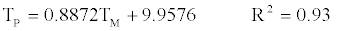(23) Where: TP is the predicted pile temperature, °C TM is the measured pile temperature, °C Conclusion A mathematical model for the compost system was developed successively according to heat balance to optimize the main factors affecting the performance of compost system through studying of pile temperature at different ambient temperatures and air flow rates. Also, carrying out an experiment to validate the model results through measuring: pile temperature. The most important results obtained can be summarized as follows: 1. The model showed that the net energy gained to the pile increases with increasing ambient temperature, meanwhile, the heat lost decreases with increasing ambient temperature. The ambient temperature increased from 15 to 35°C the heat gained increased from 7620.67 to 9605.16 kJ m-2 day-1 (40.91 to 50.03 % of total energy), but, heat loss decreased from 11005.05 to 9593.36 kJ m-2 day-1 (59.09 to 49.97 % of total energy). 2. The model showed that the predicted pile temperature increases with increasing the ambient temperature, where, the ambient air temperature increased from 15 to 35°C during the whole period of compost, the predicted pile temperature increased from 34.40 to 43.18°C. 3. The model showed that the predicted daily pile temperature decreases with increasing the airflow rate, where, the airflow rates increased from 0.7 to 1.5 mg air kg-1 dry matter during the whole period of compost, the predicted pile temperature decreased from 38.28 to 36.05°C. 4 The predicted pile temperature was in a reasonable agreement with the measured pile temperature with a coefficient of determination of 0.93. The average daily pile temperature ranged from 31.72 to 76.34°C, while it was from 18.00 to 71°C experimentally during the whole period. The results also agreed with those obtained by . Nomenclature m: total compost mass, kg Cp: heat capacity of compost, kJ kg-1 K-1 T: temperature of the pile, K t: time, day Qgen: generated energy by biodegradation, kJ day-1 Qsolar: generated energy by solar radiation, kJ day-1 Qevap: energy loss by water evaporation, kJ day-1 Qconv: energy loss by convection, kJ day-1 Qcond: energy loss by conduction, kJ day-1 Qrad: energy loss by radiation, kJ day-1 QD: energy gained by direct solar radiation, kJ day-1 Qreflected: solar energy reflected a way from the system, kJ day-1 Qsky: energy gained by long wave radiation from the sky, kJ day-1 hc: combustion of the compost material, kJ kg-1 md: dry matter of compost, kg K: degradation rate of compost at local temperature and moisture content, day-1 τ: day angle, radians n: day of the year (on January 1st , n = 1) Sc: solar constant, kJ day-1 D: distance from the Earth to the sun, km D0: mean distance from the Earth to the sun, km ψ: a “clearness” factor θZ: solar zenith angle, degrees n: pile’s latitude (positive for North),degrees δ: solar declination ω: hour angle, degrees ωtime: solar time, degrees LST: local standard time Lnt: longitude of the standard time meridian, degrees Lng: longitude of the pile, degrees Tair: absolute air temperature, K e: average emittance of the atmosphere n1: air flow exiting the composting mass, kg day-1 n2: air flow entering the composting mass, kgday-1 Cp1: average heat capacity of air exiting the composting mass, kJ kg-1 K-1 Cp2: average heat capacity of air entering the composting mass, kJ kg-1 K-1 Tsurf: surface temperature, K T0: standard temperature, K Tamb: ambient temperature, K U: overall heat transfer coefficient, kJ m-2 K-1 day-1 A: area of conduction and radiation heat transfer, m2 σ: Stefan–Boltzmann constant, kJ m-2 K-4 day-1 Fa: emissivity radiation factor Fb: configurational radiation factor Qicond: heat internally conducted from the core to the surface of the pile, kJ day-1 K: average thermal conductivity of compost, kJ m-1 K-1 day-1 Dx: average distance from the core to the surface, m FWVS: flux of vapor coming out from the material, kg day-1 Levap: latent heat of evaporation, kJ/kg water evaporated VS: vapor content in saturated gas, kg water/kg dry air TP: predicted temperature, °C TM: measured temperature, °C References Haug RT (1993) The Practical Handbook of Compost Engineering. Lewis Publishers, Boca Raton, FL. Wan NK, Hwang EY (1997) Opeational parameters for composting night soil in Korea. Compost Sci Utilization 5: 46-51. Seki H (2002) A new deterministic model for forced aeration composting processes with batch operation. Trans ASAE 45:1239-1250. Turner C1, Williams A, White R, Tillett R (2005) Inferring pathogen inactivation from the surface temperatures of compost heaps. Bioresour Technol 96: 521-529. Sommer SG, McGinn SM, Hao X, Larney FJ (2004) Techniques for measuring gas emissions from a composting stockpile of cattle manure. Atmos Environ 38: 4643-4652. Tremier A, de Guardia A, Massiani C, Paul E, Martel JL (2005) A respirometric method for characterising the organic composition and biodegradation kinetics and the temperature influence on the biodegradation kinetics, for a mixture of sludge and bulking agent to be cocomposted. Bioresour Technol 96: 169-180. Avnimelech Y, Eilat R, Porat Y, Kottas PA (2004) Factors affecting the rate of windrow composting in field studies. Compos Sci Util 12: 114-118. Bailey JE, Ollis DF (1986) Biochemical Engineering Fundamentals. McGraw-Hill. Bach PD, Nakasaki K, Shoda M, Kubota H (1987) Thermal balance in composting operations. Journal of Fermentation Technology 65: 199-209. Harper E, Miller FC, Macauley BJ (1992) Physical management and interpretation of an environmentally controlled composting ecosystem. Aust J Exp Agric 32: 657-667. Kaiser J (1996) Modelling composting as a microbial ecosystem: a simulation approach. Ecol Modell 91: 25-37. Vander Gheynst JS, Walker LP, Parlange JY (1997) Energy transport in a high solids aerobic degradation process: mathematical modeling and analysis. Biotechnol Progress 13: 238-248. Weppen P (2001) Process calorimetry on composting of municipal organic wastes. Biomass Bioenergy 21: 289-299. Ekinci K, Keener HM, Elwell DL, Michel FC (2005) Effects of aeration strategies on the composting process: Part II. Numerical modeling and simulation. Transactions of the ASAE 48: 1203-1215. Ahn HK, Richard TL, Choi HL (2007) Mass and thermal balance during composting of a poultry manure – Wood shavings mixture at different aeration rates. Process Biochem 42: 215-223. de Guardia A, Petiot C, Benoist JC, Druilhe C (2012) Characterization and modelling of the heat transfers in a pilot-scale reactor during composting under forced aeration. Waste Manag 32: 1091-1105. Mason IG (2006) Mathematical modelling of the composting process: a review. Waste Manag 26: 3-21. Robinzon R, Kimmel E, Avnimelech Y (2000) Energy and mass balances of windrow composting system. Transactions of ASAE, 43 : 1523-1529. Moraga NO, Corvalan F, Escudey M, Arias A, Zambra CE (2009) Unsteady 2D coupled heat and mass transfer in porous media with biological and chemical heat generations. Int J Heat Mass Transf 52: 5841-5848. Das K, Keener HM (1997) Numerical model for the dynamic simulation of a large scale composting system. Transactions of the ASAE, 40: 1179-1189. Keener HM, Marugg C, Hansen RC, Hoitink HA (1993) Optimizing the efficiency of the composting process. In Science and Engineering of Composting: Design Environmental, Microbiological, and Utilization Aspects. Worthington, Ohio: Renaissance Publications. Holman JP (1997) Heat Transfer (Eighth Edition). McGraw-Hill. Anderson EE (1983) Fundamentals of Solar Energy Conversion. Addison-Wesley Publishing Company. Al-ajmi FF, Loveday DL, Bedwell KH, Havenith G (2008) Thermal insulation and clothing area factors of typical Arabian Gulf clothing ensembles for males and females: measurements using thermal manikins. Appl Ergon 39: 407-414. Bliss RW (1961) Atmospheric Radiation near the Surface of the Ground. Solar Energy 5:103-120. Kondratyev YK (1969) Radiation in the Atmosphere. Academic Press. Barrena R, Canovas C, Sánchez A (2006) Prediction of temperature and thermal inertia effect in the maturation stage and stockpiling of a large composting mass. Waste Manag 26: 953-959. Barrington S, Choinière D, Trigui M, Knight W (2003) Compost convective airflow under passive aeration. Bioresour Technol 86: 259-266. Petiot C, de Guardia A (2004) Composting in a laboratory reactor: a review. Compos Sci Util 12: 69-79. Petric I, Selimbašic V (2008) Development and validation of mathematical model for aerobic composting process. Compost Sci Util 139: 304-317.

#### Tables and Figures at a glanceTable 1 Table 2 Table 3 Table 4

#### Figures at a glance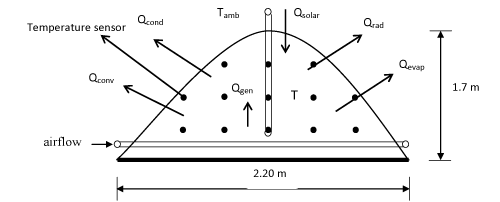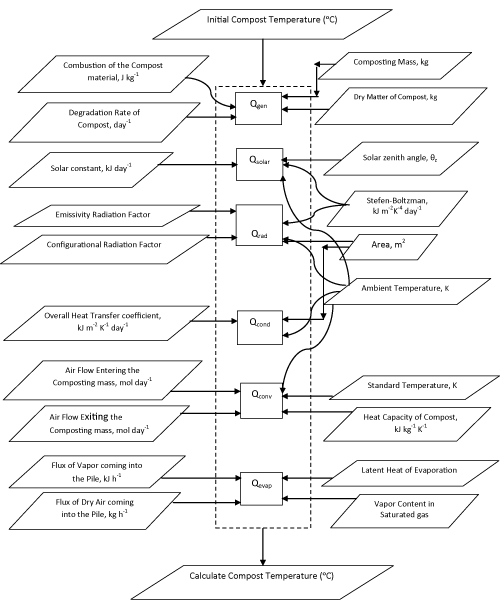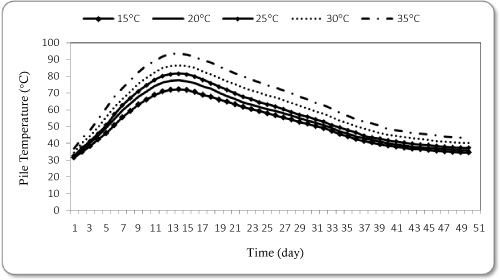Figure 1 Figure 2 Figure 3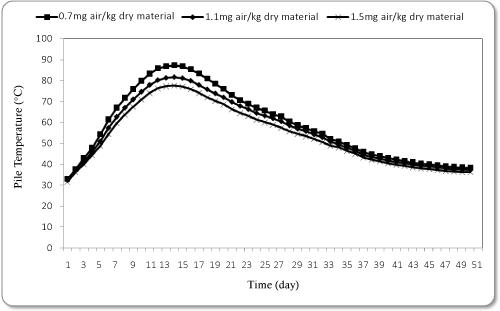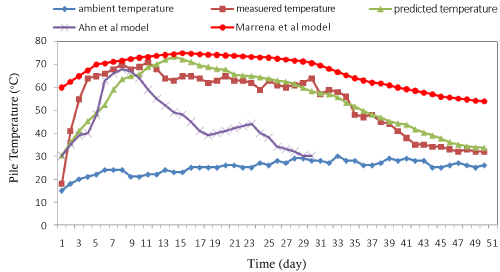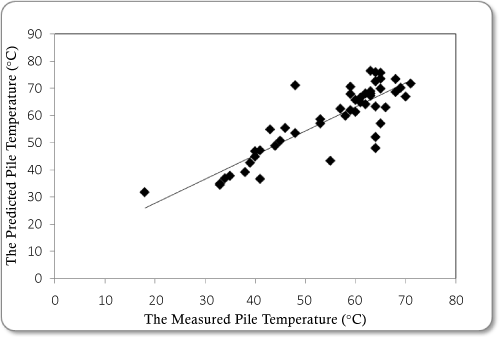Figure 4 Figure 5 Figure 6
Select your language of interest to view the total content in your interested language

### Article Usage

• Total views: 13075
• [From(publication date):
November-2014 - Dec 08, 2019]
• Breakdown by view type
• HTML page views : 9142Can't read the image? click here to refresh